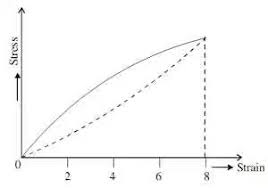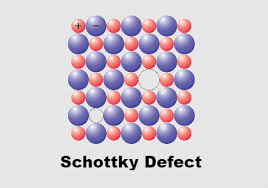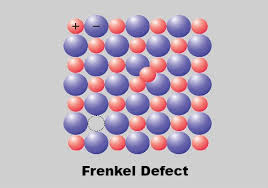## How to Calculate and Solve for Shear Modulus of Rubber | Fracture MechanicsThe image above represents shear modulus of rubber.

To compute for shear modulus of rubber, three essential parameters are needed and these parameters are Density of Network Cross Links (N), Boltzmann’s Constant (KBand Temperature (T).

The formula for calculating shear modulus of rubber:

G = NKBT

Where:

G = Shear Modulus of Rubber
N = Density of Network Cross Links
KB = Boltzmann’s Constant
T = Temperature

Let’s solve an example;
Find the shear modulus of rubber when the density of network cross links is 21, the boltzmann’s constant is 1.38064852E-23 and the temperature is 10.

This implies that;

N = Density of Network Cross Links = 21
KB = Boltzmann’s Constant = 1.38064852E-23
T = Temperature = 10

G = NKBT
G = (21)(1.38064852e-23)(10)
G = 2.89

Therefore, the shear modulus of rubber is 2.89e-23 Pa.

Calculating the Density of Network Cross Links when the Shear Modulus of Rubber, Boltzmann’s Constant and the Temperature is Given.

N = G / KBT

Where;

N = Density of Network Cross Links
G = Shear Modulus of Rubber
KB = Boltzmann’s Constant
T = Temperature

Let’s solve an example;
Find the density of network cross links when the shear modulus of rubber is 14, the boltzmann’s constant is 1.380e-23 and temperature is 4.

This implies that;

G = Shear Modulus of Rubber = 14
KB = Boltzmann’s Constant = 1.380e-23
T = Temperature = 4

N = G / KBT
N = 14 / 1.380e-23 x 4
N = 14 / 5.52e-23
N = 2.54e-23

Therefore, the network cross links is 2.54e-23.

## How to Calculate and Solve for Schottky Defect | CeramicsThe image above represents schottky defect.

To compute for schottky defect, four essential parameters are needed and these parameters are N, Activation energy (Qs), Boltzmann’s Constant (K) and Temperature (T).

The formula for calculating schottky defect:

Ns = N exp (-Qs/2KT)

Where:

Qs = Activation Energy
K = Boltzmann’s Constant
T = Temperature

Let’s solve an example;
Find the schottky defect when the activation energy is 44, N is 22, boltzmann’s constant is 1.38064852E-23 and the temperature is 30.

This implies that;

N = 22
Qs = Activation Energy = 44
K = Boltzmann’s Constant = 1.38054852E-23
T = Temperature = 30

Ns = N exp (-Qs/2KT)
Ns = (22)exp(-(44)/2(1.38064852e-23)(30))
Ns = (22)exp((-44)/(8.283891119e-22))
Ns = (22)exp(-5.3115135583771414e+22)
Ns = (22)(0)
Ns = 0

Therefore, the schottky defect is 0.

Calculating the N when the Schottky Defect, the Activation Energy, the Boltzmann’s Constant and the Temperature is Given.

N = Ns / e (-Qs / 2KT)

Where;

Ns = Schottky Defect
Qs = Activation Energy
K = Boltzmann’s Constant
T = Temperature

Let’s solve an example;
Find the N when the schottky defect is 40, the activation energy is 24, the boltzmann’s constant is 1.38064852E-23 and the temperature is 10.

This implies that;

Ns = Schottky Defect = 40
Qs = Activation Energy = 24
K = Boltzmann’s Constant = 1.38064852E-23
T = Temperature = 10

N = Ns / e (-Qs / 2KT)
N = 40 / e (-24 / 2 x 1.38064852E-23 x 10)
N = 40 / e (-24 / 2.76129704E+23)
N = 40 / e (8.691567e-23)
N = 40 / 8.691567e+23
N = 4.602e-23

Therefore, the is 4.602e-23.

## How to Calculate and Solve for Frenkel Defect | CeramicsThe image above represents frenkel defect.

To compute for frenkel defect, four essential parameters are needed and these parameters are N, activation energy (Qfr), Boltzmann’s Constant (K) and temperature (T).

The formula for calculating the frenkel defect:

Nfr = N exp (-Qfr / 2KT)

Where:

Nfr = Frenkel Defect
Qfr = Activation Energy
K = Boltzmann’s Constant
T = Temperature

Let’s solve an example;
Find the frenkel defect when the activation energy is 34, N is 22, Temperature is 12 and the boltzmann’s constant is 1.38064852e-23.

This implies that;

Qfr = Activation Energy
K = Boltzmann’s Constant
T = Temperature

Nfr = N exp (-Qfr / 2KT)
Nfr = (22)exp(-(34) / 2(1.38064852e-23)(14))
Nfr = (22)exp((-34) / (3.865815856e-22))
Nfr = (22)exp(-8.795038684325786e+22)
Nfr = (22)(0)
Nfr = 0

Therefore, the frenkel defect is 0.

Calculating the Activation Energy when the Frenkel Defect, the Boltzmann’s Constant and the Temperature is Given.

Qfr = – (In (Nfr / N) x 2KT)

Where;

Qfr = Activation Energy
Nfr = Frenkel Defect
K = Boltzmann’s Constant
T = Temperature

Let’s solve an example;
Given that the frenkel defect is 20, the boltzmann’s constant is 5, the temperature is 2 and N is 10. Find the activation energy?

This implies that;

Nfr = Frenkel Defect = 20
K = Boltzmann’s Constant = 5
T = Temperature = 2
N = 10

Qfr = – (In (Nfr / N) x 2KT)
Qfr = – (In (20 / 10) x 2 x 5 x 2)
Qfr = – (In 2 x 20)
Qfr = – (In 40)
Qfr = – 3.688

Therefore, the activation energy is – 3.688.